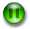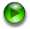# About the Longitudinal Wave Animation

This animation illustrates a longitudinal wave, such as a sound wave travelling through the air.

The red spheres at the bottom represent the air molecules. Each molecule is executing simple harmonic motion about its equilibrium position. The equilibrium position of each molecule is represented by a vertical black line.

As a molecule oscillates it pushes and pulls its neighbor to the right, which then executes the same simple harmonic motion but with a time delay. This molecule in turn pushes and pulls its neighbor to the right, so the oscillations propagate through the air.

The displacement of each molecule from its equilibrium position is also shown by the black circles at the top of the animation. Here the equilibrium positions are represented by the horizontal black line. From this part of the animation, you can see that the displacements form a wave shaped like a sine function that is propagating to the right.

This wave is called a displacement wave since it is a wave in the displacements of the molecules from their equilibrium positions.

There are four controls for the animation, located in the lower left corner:Causes the animation to "step" back one frameCauses the animation to "step" forward one framePauses the animationPlays the animation

If you use these controls to pause the animation when molecule 3 is at its equilibrium position and look at the molecule and its nearest neighbors you will see that the density of molecules in that region is either a minimum or a maximum. This means that the pressure of the air in that region is also a minimum or a maximum, since the pressure is proportional to the density of molecules.

When molecule 3 is at its equilibrium position, so is molecule 9. If the density around molecule 3 is a minimum the density around molecule 9 is a maximum, and vice versa.

Meanwhile, molecule 6 is at its maximum or minimum displacement, and the density of molecules at that position is the average value. Thus, the pressure in that region is just atmospheric pressure.

In fact, we can also think about the sound wave being a pressure wave, that is also shaped like a sine wave and is travelling from the left to the right. The pressure varies about atmospheric pressure, so atmospheric pressure corresponds to zero amplitude in the pressure wave.

However, as we have seen, when the displacement wave is a minimum or maximum, the pressure wave is zero. When the displacement wave is zero, the pressure wave is a minimum or maximum. So these two waves are 90 degrees out of phase with each other.